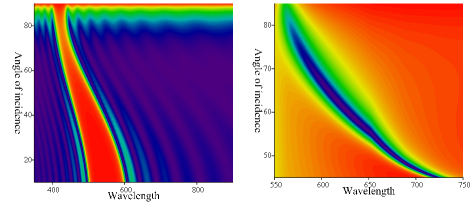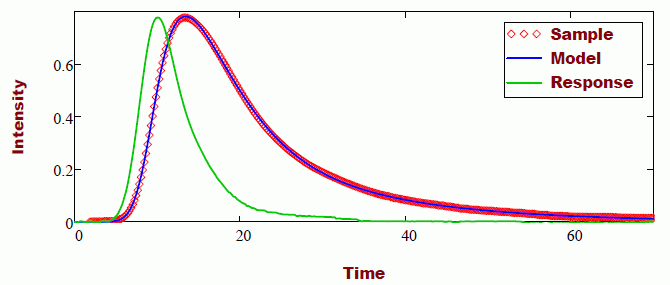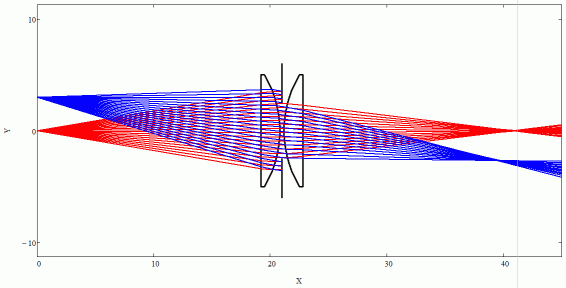﻿ Valter Kiisk » Mathcad worksheets

This page lists a number of (rather old) Mathcad worksheets developed for scientific data analysis or educational purposes. Although the calculations are in some cases quite simple, the main peculiarity of the worksheets is an attempt to realize a convenient and clean user interface for the calculations (within the confines of Mathcad programming capabilities). The calculation management (data input, output, settings) is automated as much as possible whereas all internal complexity of the calculation is hidden inside collapsible areas.

These worksheets have been developed and tested using Mathcad 15.

## Specialty scientific programs

### Deconvolution of thermoluminescence glow curves

The program fits arbitrary glow peak models to an experimental TL glow curve. The program is described by an article in Radiation Protection Dosimetry (10.1093/rpd/nct072).

Preview### Simulation of thermoluminescence glow curves

The program simulates the TL glow curve (as well as phosphorescence afterglow, etc) of arbitrary interactive system of traps and recombination centers. The program is described by an article in Radiation Protection Dosimetry (doi 10.1093/rpd/nct072).

Preview

### Optics of planar layered structure

The program evaluates the reflectance or transmittance spectrum of arbitrary planar layered structure as a function of wavelength and the angle of incidence. The materials are assumed to be non-birefringent but may expose an absorbance (i.e. the refractive index is allowed to be complex). The program can be used to simulate interference filters, plasmonic structures, etc.

Preview### Analysis of thin film transmittance

The program calculates the thickness and the optical constants of a thin film on a transparent substrate from the transmission spectrum based on the method and formulae by R. Swanepoel [J. Phys. E 16 (1983), 1214-1222]. It contains both the envelope method as well as least-squares fitting (the latter requires the use of a dispersion model).

Preview### Combined peak-fitting of multiple spectra

The program performs simultaneous peak-fitting of multiple spectra.

Preview

### Fit a decay curve with a distribution of decay rates

The program performs a least-squares fitting of decaying signals (e.g. fluorescence decay) with various decay models based on a continuous distribution of decay constants (such as Förster or Becquerel decay functions).

Preview### Deconvolution of decay kinetics against instrumental response

The program fits an experimental decay curve (e.g. fluorescence decay measured in time domain) by (multi)exponential decay convoluted with the response function of the system recorded under identical conditions (e.g. the shape of the excitation pulse in the case of fluorescence measurement). It can either (a) model the response function by an asymmetric Gaussian or (b) use FFT to directly convolute the response function and the decay model.

Preview### Digitizer

This is yet another program that tries to recover plotted data from a bitmap image.

Preview

## Educational programs

### 2D optical ray tracer

The program carries out an exact tracing of meridional optical rays through an axially symmetric optical system consisting of spherical, planar or parabolic refracting surfaces, and apertures.